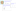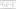Please scroll down to see the Georgia Standards of Excellence recommended curriculum for 6th grade math.

Please scroll down to see the Georgia Standards of Excellence recommended curriculum for 6th grade math.

## NS – The Number System

Apply and extend previous understandings of multiplication and division to divide fractions by fractions.

MGSE6.NS.1 Interpret and compute quotients of fractions, and solve word problems involving division of fractions by fractions, including reasoning strategies such as using visual fraction models and equations to represent the problem.

For example:

• How much chocolate will each person get if 3 people share 1/2 lb of chocolate equally?
• How many 3/4-cup servings are in 2/3 of a cup of yogurt?
• How wide is a rectangular strip of land with length 3/4 mi and area 1/2 square mi?
• Create a story context for (2/3)÷(3/4)and use a visual fraction model to show the quotient;
• Three pizzas are cut so each person at the table receives 1⁄4 pizza. How many people are at the table?
• Use the relationship between multiplication and division to explain that (2/3)÷(3/4)= 8/9 because 3/4 of 8/9 is2/3. (In general, (a/b)÷(c/d) =ad/bc.)

Compute fluently with multi-digit numbers and find common factors and multiples.

MGSE6.NS.2 Fluently divide multi-digit numbers using the standard algorithm.

MGSE6.NS.3 Fluently add, subtract, multiply, and divide multi-digit decimals using the standard algorithm for each operation.

MGSE6.NS.4 Find the common multiples of two whole numbers less than or equal to 12 and the common factors of two whole numbers less than or equal to 100.

a. Find the greatest common factor of 2 whole numbers and use the distributive property to express a sum of two whole numbers 1-100 with a common factor as a multiple of a sum of two whole numbers with no common factors. (GCF).

For example : 36 + 8 = 4(9 + 2).

b. Apply the least common multiple of two whole numbers less than or equal to 12 to solve real-world problems.

Apply and extend previous understandings of numbers to the system of rational numbers.

MGSE6.NS.5 Understand that positive and negative numbers are used together to describe quantities having opposite directions or values (e.g.,temperature above/below zero, elevation above/below sea level, debits/credits, positive/negative electric charge); use positive and negative numbers to represent quantities in real-world contexts, explaining the meaning of 0 in each situation.

MGSE6.NS.6 Understand a rational number as a point on the number line. Extend number line diagrams and coordinate axes familiar from previous grades to represent points on the line and in the plane with negative number coordinates.

MGSE6.NS.6a Recognize opposite signs of numbers as indicating locations on opposite sides of 0 on the number line; recognize that the opposite of the opposite of a number is the number itself, e.g., –(–3) = 3, and that 0 is its own opposite.

MGSE6.NS.6b Understand signs of numbers in ordered pairs as indicating locations in quadrants of the coordinate plane; recognize that when two ordered pairs differ only by signs, the locations of the points are related by reflections across one or both axes.

MGSE6.NS.6c Find and position integers and other rational numbers on a horizontal or vertical number line diagram; find and position pairs of integers and other rational numbers on a coordinate plane.

MGSE6.NS.7 Understand ordering and absolute value of rational numbers.

MGSE6.NS.7a Interpret statements of inequality as statements about the relative position of two numbers on a number line diagram.

MGSE6.NS.7b Write, interpret, and explain statements of order for rational numbers in real-world contexts.

MGSE6.NS.7c Understand the absolute value of a rational number as its distance from 0 on the number line; interpret absolute value as magnitude for a positive or negative quantity in a real-world situation.

MGSE6.NS.7d Distinguish comparisons of absolute value from statements about order.

MGSE6.NS.8 Solve real-world and mathematical problems by graphing points in all four quadrants of the coordinate plane. Include use of coordinates and absolute value to find distances between points with the same first coordinate or the same second coordinate.

## RP – Ratios and Proportional Relationships

Understand ratio concepts and use ratio reasoning to solve problems.

MGSE6.RP.1 Understand the concept of a ratio and use ratio language to describe a ratio relationship between two quantities.

For example, “The ratio of wings to beaks in the bird house at the zoo was 2:1, because for every 2 wings there was 1 beak.” “For every vote candidate A received, candidate C received nearly three votes.”

MGSE6.RP.2 Understand the concept of a unit rate a / b associated with a ratio a: b with b ≠ 0 (b not equal to zero), and use rate language in the context of a ratio relationship.

For example, "This recipe has a ratio of 3 cups of flour to 4 cups of sugar, so there is 3/4 cup of flour for each cup of sugar." "We paid \$75 for 15 hamburgers, which is a rate of \$5 per hamburger."

MGSE6.RP.3 Use ratio and rate reasoning to solve real-world and mathematical problems utilizing strategies such as tables of equivalent ratios, tape diagrams (bar models), double number line diagrams, and/or equations.

MGSE6.RP.3a Make tables of equivalent ratios relating quantities with whole-number measurements, find missing values in the tables, and plot the pairs of values on the coordinate plane. Use tables to compare ratios.

MGSE6.RP.3b Solve unit rate problems including those involving unit pricing and constant speed.

For example, If it took 7 hours to mow 4 lawns, then at that rate, how many lawns could be mowed in 35 hours? At what rate were lawns being mowed?

MGSE6.RP.3c Find a percent of a quantity as a rate per 100 (e.g. 30% of a quantity means 30/100 times the quantity); given a percent, solve problems involving finding the whole given a part and the part given the whole.

MGSE6.RP.3d Given a conversion factor, use ratio reasoning to convert measurement units within one system of measurement and between two systems of measurements (customary and metric); manipulate and transform units appropriately when multiplying or dividing quantities.

For example, given 1 in. = 2.54 cm, how many centimeters are in 6 inches?

## EE - Expressions and Equations

Apply and extend previous understandings of arithmetic to algebraic expressions.

MGSE6.EE.1 Write and evaluate numerical expressions involving whole-number exponents.

MGSE6.EE.2 Write, read, and evaluate expressions in which letters stand for numbers.

MGSE6.EE.2a Write expressions that record operations with numbers and with letters standing for numbers.

MGSE6.EE.2b Identify parts of an expression using mathematical terms (sum, term, product, factor, quotient, coefficient); view one or more parts of an expression as a single entity.

MGSE6.EE.2c Evaluate expressions at specific values for their variables. Include expressions that arise from formulas in real-world problems. Perform arithmetic operations, including those involving whole-number exponents, in the conventional order when there are no parentheses to specify a particular order (Order of Operations).

MGSE6.EE.3 Apply the properties of operations to generate equivalent expressions.

MGSE6.EE.4 Identify when two expressions are equivalent (i.e., when the two expressions name the same number regardless of which value is substituted into them).

Reason about and solve one-variable equations and inequalities.

MGSE6.EE.5 Understand solving an equation or inequality as a process of answering a question: which values from a specified set, if any, make the equation or inequality true? Use substitution to determine whether a given number in a specified set makes an equation or inequality true.

MGSE6.EE.6 Use variables to represent numbers and write expressions when solving a real-world or mathematical problem; understand that a variable can represent an unknown number, or, depending on the purpose at hand, any number in a specified set.

MGSE6.EE.7 Solve real-world and mathematical problems by writing and solving equations of the form x _+p _=q and px _= q _for cases in which p, q and x are all non negative rational numbers.

MGSE6.EE.8 Write an inequality of the form x>_c _or x<_ c_to represent a constraint or condition in a real-world or mathematical problem. Recognize that inequalities of the form x>_c _or x<_c _have infinitely many solutions; represent solutions of such inequalities on number line diagrams.

Represent and analyze quantitative relationships between dependent and independent variables.

MGSE6.EE.9 Use variables to represent two quantities in a real-world problem that change in relationship to one another.

a. Write an equation to express one quantity, the dependent variable, in terms of the other quantity, the independent variable.

b. Analyze the relationship between the dependent and independent variables using graphs and tables, and relate these to the equation.

For example, in a problem involving motion at constant speed, list and graph ordered pairs of distances and times, and write the equation d=65t to represent the relationship between distance and time.

## G – Geometry

Solve real-world and mathematical problems involving area, surface area, and volume.

MGSE6.G.1 Find area of right triangles, other triangles, quadrilaterals, and polygons by composing into rectangles or decomposing into triangles and other shapes; apply these techniques in the context of solving real-world and mathematical problems.

MGSE6.G.2 Find the volume of a right rectangular prism with fractional edge lengths by packing it with unit cubes of the appropriate unit fraction edge lengths (1/2 u), and show that the volume is the same as would be found by multiplying the edge lengths of the prism. Apply the formulas V = (length) x (width) x (height) and V= (area of base) x (height) to find volumes of right rectangular prisms with fractional edge lengths in the context of solving real-world and mathematical problems.

MGSE6.G.3 Draw polygons in the coordinate plane given coordinates for the vertices; use coordinates to find the length of a side joining points with the same first coordinate or the same second coordinate. Apply these techniques in the context of solving real-world and mathematical problems.

MGSE6.G.4 Represent three-dimensional figures using nets made up of rectangles and triangles, and use the nets to find the surface area of these figures. Apply these techniques in the context of solving real-world and mathematical problems.

## SP – Statistics and Probability

Develop understanding of statistical variability.

MGSE6.SP.1 Recognize a statistical question as one that anticipates variability in the data related to the question and accounts for it in the answers.

MGSE6.SP.2 Understand that a set of data collected to answer a statistical question has a distribution which can be described by its center, spread, and overall shape.

MGSE6.SP.3 Recognize that a measure of center for a numerical data set summarizes all of its values with a single number, while a measure of variation describes how its values vary with a single number. Summarize and describe distributions.

MGSE6.SP.4 Display numerical data in plots on a number line, including dot plots, histograms, and box plots.

MGSE6.SP.5 Summarize numerical data sets in relation to their context, such as by:

a. Reporting the number of observations.

b. Describing the nature of the attribute under investigation, including how it was measured and its units of measurement.

c. Giving quantitative measures of center (median and/or mean) and variability (interquartile range).

d. Relating the choice of measures of center and variability to the shape of the data distribution and the context in which the data was gathered.